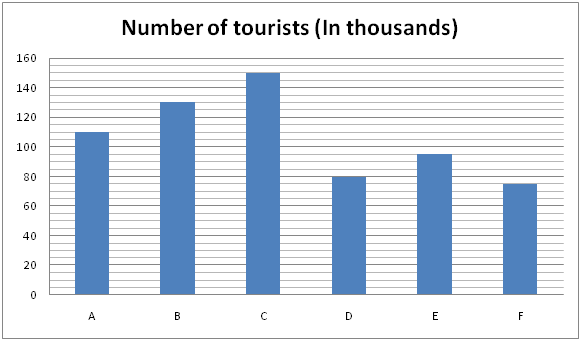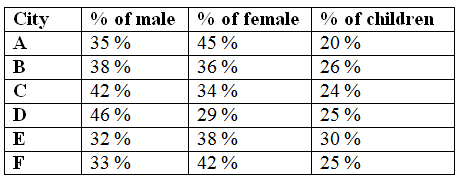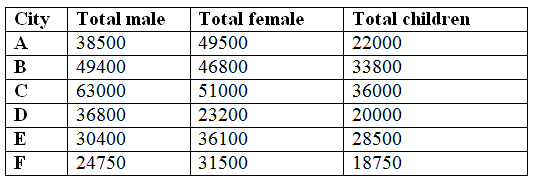# NIACL AO Mains– Quantitative Aptitude Questions Day- 18

Dear Readers, Bank Exam Race for the Year 2019 is already started, To enrich your preparation here we have providing new series of Practice Questions on Quantitative Aptitude – Section. Candidates those who are preparing for NIACL AO Mains 2019 Exams can practice these questions daily and make your preparation effective.

[WpProQuiz 5250]

Directions (Q. 1 – 5) In the following questions, two equations I and II are given. You have to solve both the equations and give answer as,

a) If x > y

b) If x ≥ y

c) If x < y

d) If x ≤ y

e) If x = y or the relation cannot be established

1) I) x2 – 9x – 252 = 0

II) y2 – 6y – 187 = 0

2) I) 3/√x + 7/√x = √x

II) y2 = 125/2/ √y

3) I) 3x = 5y – 4

II) 5x + 7y – 70 = 0

4) I) 4x2 + 4x – 48 = 0

II) 3y2 – 22y + 32 = 0

5) I) x2 + 24x – 81 = 0

II) y2 + y – 56 = 0

Directions (Q. 6 – 10) Study the following information carefully and answer the given questions:

The following bar graph shows the number of tourists (in thousands) visited six different places in India in the year 2016 and the table shows the percentage of male, female and children’s among them.6) Find the total number of male tourists visited all the given cities together?

a) 242850

b) 256730

c) 198640

d) 295460

e) None of these

7) Total number of females and children’s who are all visited the city A and D together is approximately what percentage more/less than the total number of males and children’s who are all visited the city B and E together?

a) 20 % more

b) 30 % more

c) 10 % more

d) 20 % less

e) 30 % less

8) Find the difference between the total number of males who are all visited the city B, C and F together to that of total number of females who are all visited the city A, D and E together?

a) 19870

b) 21740

c) 28350

d) 27560

e) None of these

9) Find the ratio between the total number of males to that of children’s who are all visited all the given cities together?

a) 4112 : 2895

b) 4857 : 3181

c) 3562 : 2893

d) 5126 : 3895

e) None of these

10) Total number of peoples who visited the city A, B and E together is approximately what percentage of the total number of peoples who visited the city C, D and F together?

a) 110 %

b) 125 %

c) 95 %

d) 80 %

e) 135 %

Direction (1-5) :

I) x2 – 9x – 252 = 0

(x – 21) (x + 12) = 0

X = 21, -12

II) y2 – 6y – 187 = 0

(y – 17) (y + 11) = 0

Y = 17, -11

Can’t be determined

I) 3/√x + 7/√x = √x

(3 + 7) / √x = √x

X = 10

II) y2 = 125/2/ √y

y2 × √y = 125/2

y2 + (1/2) = 125/2

y5/2 = 125/2

y = 12

x < y

3x – 5y = 4–> (1)

5x + 7y = 70–>(2)

By solving the equation (1) and (2),

X = 7, y = 5

x > y

I) 4x2 + 4x – 48 = 0

4x2 + 16x – 12x – 48 = 0

4x (x + 4) – 12 (x + 4) = 0

(4x – 12) (x + 4) = 0

X = 12/4, -4 = 3, -4

II) 3y2 – 22y + 32 = 0

3y2 – 6y – 16y + 32 = 0

3y (y – 2) – 16 (y – 2) = 0

(3y – 16) (y – 2) = 0

Y = 16/3, 2 = 5.33, 2

Can’t be determined

I) x2 + 24x – 81 = 0

(x + 27) (x – 3) = 0

X = -27, 3

II) y2 + y – 56 = 0

(y + 8) (y – 7) = 0

Y = -8, 7

Can’t be determinedThe total number of male tourists visited all the given cities together

= > 38500 + 49400 + 63000 + 36800 + 30400 + 24750

= > 242850

Total number of females and children’s who are all visited the city A and D together

= > 49500 + 22000 + 23200 + 20000

= > 114700

Total number of males and children’s who are all visited the city B and E together

= > 49400 + 33800 + 30400 + 28500

= > 142100

Required % = [(142100 – 114700)/142100]*100

= > 19.28 % = 20 % less

The total number of males who are all visited the city B, C and F together

= > 49400 +63000 + 24750 = 137150

The total number of females who are all visited the city A, D and E together

= > 49500 + 23200 + 36100 = 108800

Required difference = 137150 – 108800 = 28350

The total number of males who are all visited all the given cities together

= > 38500 + 49400 + 63000 + 36800 + 30400 + 24750

= > 242850

The total number of children’s who are all visited all the given cities together

= > 22000 + 33800 + 36000 + 20000 + 28500 + 18750

= > 159050

Required ratio = 242850 : 159050 = 4857 : 3181

Total number of peoples who visited the city A, B and E together

= > 110000 + 130000 + 95000

= > 335000

Total number of peoples who visited the city C, D and F together

= > 150000 + 80000 + 75000

= > 305000

Required % = (335000/305000)*100 = 110 %